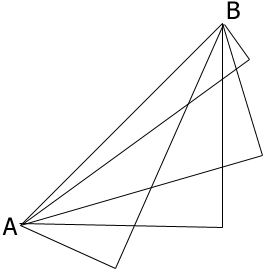SEARCH HOMEMath Central Quandaries & QueriesQuestion from Jeevan, a student: how can i find the height and base of a right angle triangle if i have the hypotenuse only ?You don't. Below is a diagram showing a hypotenuse $AB$ and 4right triangles with different heights and bases and all with $AB$ as the hypotenuse.PennyMath Central is supported by the University of Regina and The Pacific Institute for the Mathematical Sciences.